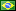###Author Topic: Input integers to Intel x86 NASM through C  (Read 4080 times)

####ImConfussed

• Jr. Member
•• Posts: 2##### Input integers to Intel x86 NASM through C
« on: February 08, 2020, 10:45:33 PM »
Hello. I have an assignment where I have to calculate the determinant of a matrix on Intel x86 with NASM, but I have to take the elements of the matrix and show the final result using C.
My problem: I (and all my classmates) started from 0 with NASM, and all we have is Paul Carter's PC Assembly book.

I managed to do an incredible poor .asm where I ask for 4 inputs, store each one of them on 4 variables declared on the .bss section and then do the math (as if those for numbers where the elements of a 2x2 matrix).

Carter's book goes from easy to hard in no time. I can not do a simple program where I ask for 2 numbers in C (using scanf("%d %d", &num1, &num2)), add them on NASM and return the value to C to show it.

My C code is simple:
Code: [Select]
`#include <stdio.h>void calc_sum(int, int, int*) __attribute__((cdecl));int main(void){    int num1, num2, sum;    printf("Input two numbers: ");    scanf("%d %d", &num1, &num2);    calc_sum(num1, num2, &sum);          // here the .asm does result = num1 + num2 and returns the result    printf("Sum is %d\n", sum);}`
(if there is a typo is because I'm retyping it from my VM with Ubuntu 18.04 to Windows)

Someone knows of a guide o a place with basics examples, or can help me with this (not the determinant, but implementing this C code in a .asm)?

####ImConfussed

• Jr. Member
•• Posts: 2##### Re: Input integers to Intel x86 NASM through C
« Reply #1 on: February 10, 2020, 02:27:14 PM »
Done. Maybe this is useful for someone, so:

Code: [Select]
`%include "asm_io.inc"segment .text    global calc_sumcalc_sum:    enter       4, 0    push        ebx    ;dump_stack  1, 0, 6    mov         ebx,[ebp+16]      mov         eax,[ebp+8]       ; [ebp+8] = num1    add         eax,[ebp+12]      ; [ebp+12] = num2    ; if I were to have num3, then it would be [ebp+16], and the currently [ebp+16], would now be on [ebp+20]    mov         [ebx],eax    pop         ebx    leave    ret`

####fredericopissarra

• Full Member
•• Posts: 171
• Country:##### Re: Input integers to Intel x86 NASM through C
« Reply #2 on: February 11, 2020, 09:53:17 AM »
Here's three shorter versions:

Code: [Select]
`; sum32.asm - 32 bits version (linux + windows) - cdecl.bits  32section .text; Instead of remembering where the arguments; are on the stack, you can use a structure like this:struc sumstkfrm.retaddr:   resd  1  ; return address.b:         resd  1.a:         resd  1endstruc; int sum( int a, int b );global sumsum:  mov eax, [esp + sumstkfrm.a]  add eax, [esp + sumstkfrm.b]  ret`
Code: [Select]
`; sumx86-64-linux.asm; for Linux amd64 version.bits 64section .text; int sum( int a, int b );global sumsum:  lea  eax, [rdi+rsi]  ret`
Code: [Select]
`; sumx86-64-windows.asm; for Windows x64 version.bits 64section .text; int sum( int a, int b );global sumsum:  lea  eax, [rcx+rdx]  ret`

####fredericopissarra

• Full Member
•• Posts: 171
• Country:##### Re: Input integers to Intel x86 NASM through C
« Reply #3 on: February 11, 2020, 12:23:14 PM »
Since you are using GCC, if you really need an assembly version for your sum() function, I recomend using inline assembly to avoid unecessary calls:
Code: [Select]
`// this works in every Intel/AMD processors...#define sum(s,a,b) \  __asm__ __volatile__ ( "leal (%1,%2),%0" : \    "=r" ((s)) : "r" ((a)), "r" ((b)) );...  int r, a, b;...  sum(r,a,b);`